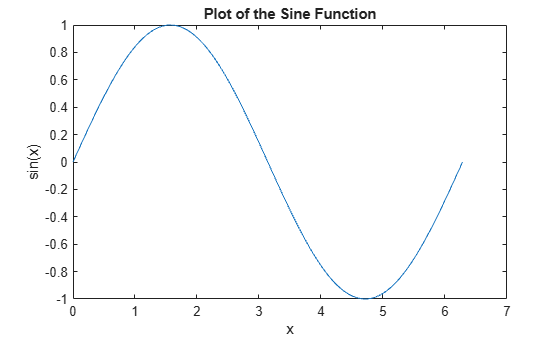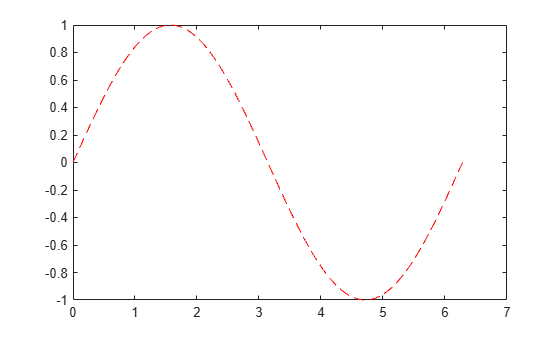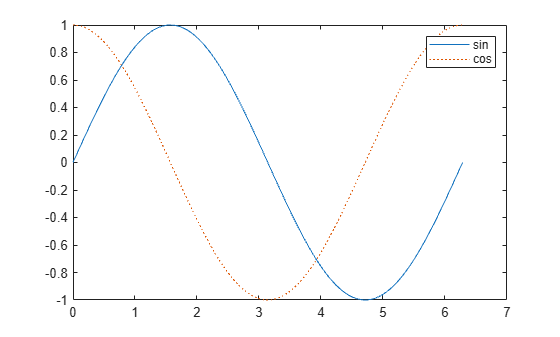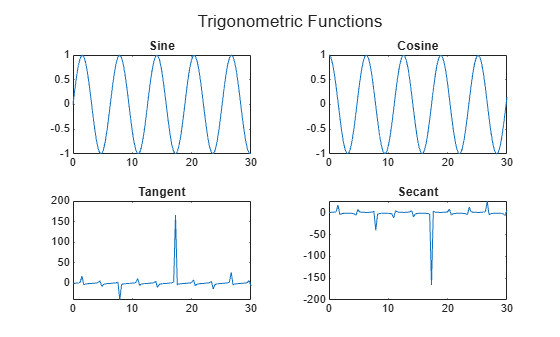Documentation

### This is machine translation

Mouseover text to see original. Click the button below to return to the English version of the page.

Note: This page has been translated by MathWorks. Click here to see
To view all translated materials including this page, select Country from the country navigator on the bottom of this page.

## 2-D and 3-D Plots

### Line Plots

To create two-dimensional line plots, use the `plot` function. For example, plot the value of the sine function from 0 to $2\pi$:

```x = 0:pi/100:2*pi; y = sin(x); plot(x,y)```You can label the axes and add a title.

```xlabel('x') ylabel('sin(x)') title('Plot of the Sine Function')```By adding a third input argument to the `plot` function, you can plot the same variables using a red dashed line.

`plot(x,y,'r--')``'r--'` is a line specification. Each specification can include characters for the line color, style, and marker. A marker is a symbol that appears at each plotted data point, such as a `+`, `o`, or `*`. For example, `'g:*'` requests a dotted green line with `*` markers.

Notice that the titles and labels that you defined for the first plot are no longer in the current figure window. By default, MATLAB® clears the figure each time you call a plotting function, resetting the axes and other elements to prepare the new plot.

To add plots to an existing figure, use `hold on`. Until you use `hold off` or close the window, all plots appear in the current figure window.

```x = 0:pi/100:2*pi; y = sin(x); plot(x,y) hold on y2 = cos(x); plot(x,y2,':') legend('sin','cos') hold off```### 3-D Plots

Three-dimensional plots typically display a surface defined by a function in two variables, z = f(x,y) .

To evaluate z, first create a set of (x,y) points over the domain of the function using `meshgrid`.

```[X,Y] = meshgrid(-2:.2:2); Z = X .* exp(-X.^2 - Y.^2);```

Then, create a surface plot.

`surf(X,Y,Z)`Both the `surf` function and its companion `mesh` display surfaces in three dimensions. `surf` displays both the connecting lines and the faces of the surface in color. `mesh` produces wireframe surfaces that color only the lines connecting the defining points.

### Subplots

You can display multiple plots in different subregions of the same window using the `subplot` function.

The first two inputs to `subplot` indicate the number of plots in each row and column. The third input specifies which plot is active. For example, create four plots in a 2-by-2 grid within a figure window.

```t = 0:pi/10:2*pi; [X,Y,Z] = cylinder(4*cos(t)); subplot(2,2,1); mesh(X); title('X'); subplot(2,2,2); mesh(Y); title('Y'); subplot(2,2,3); mesh(Z); title('Z'); subplot(2,2,4); mesh(X,Y,Z); title('X,Y,Z');```Download ebook